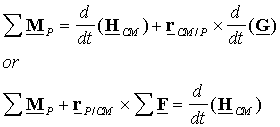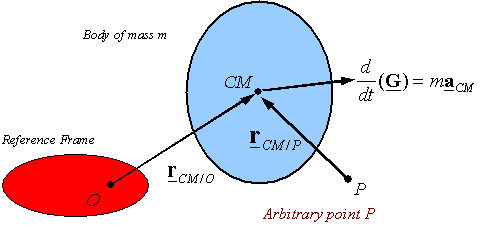Equations of motion for a rigid body

(Euler’s laws)

Inertial frame: The explicit form of the laws of mechanics depend on the frame used to reference the motions. The reference frame is frequently the background of the event, the earth being the most common reference frame. For example, Newton’s second law is written as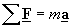when the acceleration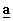is calculated relative to an inertial reference frame. The points of an inertial frame have no acceleration (purely translating with constant or zero velocity, and no rotation). On the other hand, this same law will be written as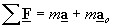if the accelerationis calculated with respect to a frame which is purely translating with an acceleration of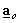. Therefore, it is important to consider the reference frame carefully before using any particular form of a given law. Most of the time, the laws of motion are written relative to an inertial frame, then from this form one can derive the form of the law relative to other frames.

Euler’s laws: The laws of motion for a rigid body are known as Euler’s laws. Euler gave two laws for the motion of a rigid body. The two laws written relative to an inertial reference frame are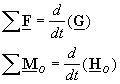where O is a fixed point on the inertial reference frame. The first of Euler’s two laws describes how the forces control the “translational” motion of the rigid body (i.e., the change of the velocity of the center of mass,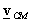). The second of Euler’s two laws describes how the change of angular momentum of the rigid body is controlled by the moment of forces and couples applied on the body. The laws of Euler are written for a body of fixed matter (i.e., matter can not be added to the body, matter can not be subtracted from the body, nor can matter be replaced by other matter).

An alternate form of these laws which replaces the need to have a fixed point O on an inertial frame is obtained by using the center of mass. This law is  derived below and given by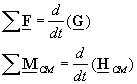where CM stands for the center of mass.

A third alternate form of Euler’s laws can be derived from the second alternate form. This form replaces the center of mass by an arbitrary point P which may be moving in any way (it can be accelerating). This form of Euler’s laws are written as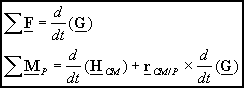where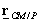is the position of the center of mass relative to the selected point P. Details of how to obtain this form is also given below. A fourth form of Euler’s laws is given aswhere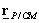is the position of point P with respect to the center of mass, and as above P may be selected arbitrarily.

It will also be shown that the linear momentum of a rigid body is given by the mass times the velocity of the center of mass of the body to give the alternate form of Euler’s first law as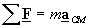where m is the total mass of the rigid body and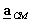is the acceleration of the center of mass of the body.

The linear momentum of a particle: For a single particle of mass m its linear momentum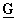is given by its mass times its velocity so that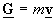The linear momentum of a body: The linear momentum of a body is assumed to be the sum of the linear momentum of its “particles”. For example, if one has a body consisting of several particles each having a mass of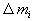and a velocity of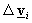, its linear momentum is given by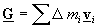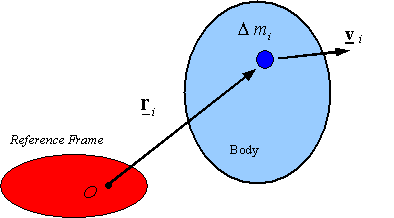It is simple to see that for a continuous body the summation can be replaced by and integration to get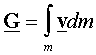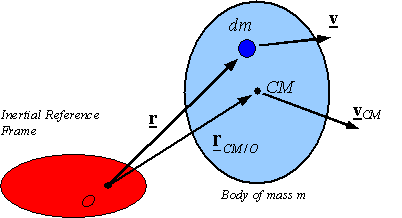The location of the center of mass of a body can be found from  the relation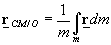Rearranging this relation and taking its time derivative relative to an inertial frame yields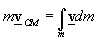whereis the velocity of the center of mass measured with respect to the reference frame and m is the total mass of the body. Therefore, one can conclude that the linear momentum of a body is given by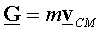Alternate form of Euler’s first law: Using the above relation for the linear momentum of a rigid body, one can replace the linear momentum by the mass times the velocity of the center of mass to obtain the alternate form of Euler’s first law as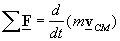Since mass is constant in Newtonian mechanics, one arrives at the relation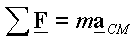Note: For a single particle the center of mass and the location of the particle are the same and one recovers Newton’s second law.

Angular momentum of a particle: The symbol HO denotes the angular momentum and for a particle of mass m is defined as the moment of linear momentum around the point O, and given by the equation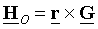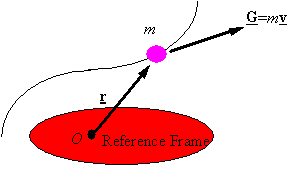Angular momentum for a rigid body: The angular momentum for a rigid body is the sum of the angular momentum for the “particles” in it. Given a reference frame, a point O on this reference frame, and a rigid body, one can approximate the angular momentum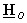of the rigid body as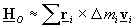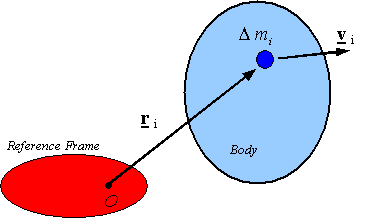where the body is assumed to be approximated by a sum of parts, each having a mass, a position, and a velocity.

Taking a limit as the parts get smaller brings one to the following integral representation of the angular momentum of a rigid bodywhere m is the total mass of the body and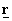and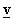are, respectively, the position and velocity of dm relative to the selected reference frame and the selected reference point O on it.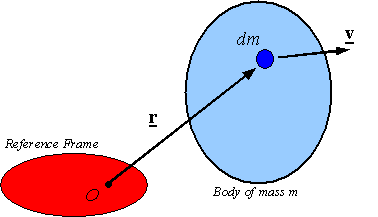The relation between moments of forces when the moment is taken about different points: The moment of a force taken about O can be related to the moment of the same force taken about point A by the relation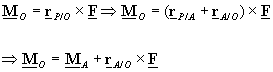The relation between angular momentums when the angular momentum is taken about different points: The angular momentum of a body taken about O can be related to the angular momentum of the same body taken about point A by the relation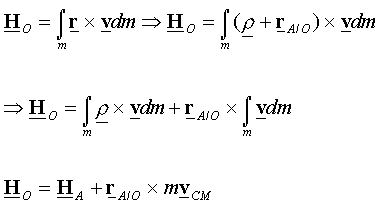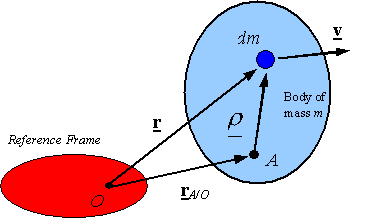Alternate forms of Euler’s second law: A useful alternate form to Euler’s second law can be obtained by using the above change of reference point rules to write the second law as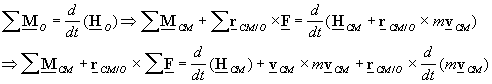Taking advantage of the Euler’s first law, and noting that the second term on the right hand side is zero, one gets the alternate form of Euler’s second law as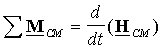The importance of this form is that the moment and the angular momentum are calculated relative to the center of mass, which need not be on an inertial frame. Therefore, the need to use a selected point O on an inertial frame has been removed in favor of the center of mass that may be accelerating. Taking the moment of Euler’s first law around an arbitrary point P and adding it to this equation results in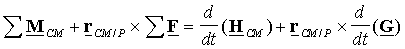One can rewrite this in one of the two other alternate forms